Homework Help Question & Answers

# I NEED HELP~ ACTIVITY 4 Activity 4: Newton's Law of Gravity: The force of gravity between...

I NEED HELP~ ACTIVITY 4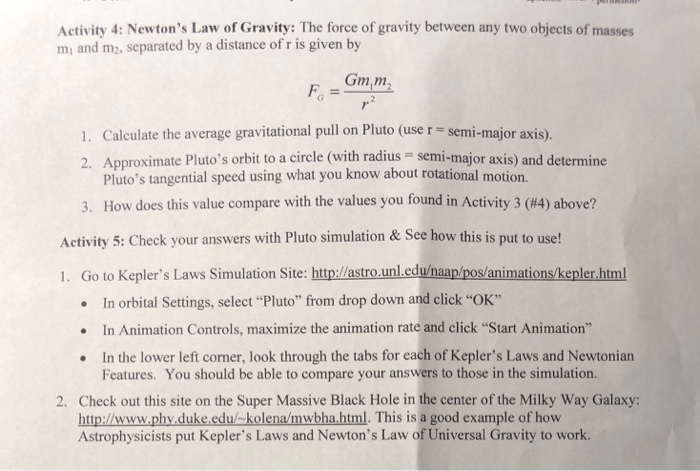Activity 4: Newton's Law of Gravity: The force of gravity between any two objects of masses mi and m2, separated by a distance of r is given by Gmm 1. Calculate the average gravitational pull on Pluto (use r - semi-major axis). 2. Approximate Pluto's orbit to a circle (with radius semi-major axis) and determine Pluto's tangential speed using what you know about rotational motion. How does this value compare with the values you found in Activity 3 (#4) above? 3, Adhit s Clecd our mnee ow n s pat e 1. Go to Kepler's Laws Simulation Site: http://astro.unleduhaap/pos/animations/kepler.html In orbital Settings, select"Pluto" from drop down and click "OK" In Animation Controls, maximize the animation rate and click "Start Animation" In the lower left corner, look through the tabs for each of Kepler's Laws and Newtonian · . . Features. You should be able to compare your answers to those in the simulation. 2. Check out this site on the Super Massive Black Hole in the center of the Milky Way Galaxy http:/www.phy duke.edu/-kolena/mwbha.html. This is a good example of how Astrophysicists put Kepler's Laws and Newton's Law of Universal Gravity to work.

#### Homework Answers

Answer #1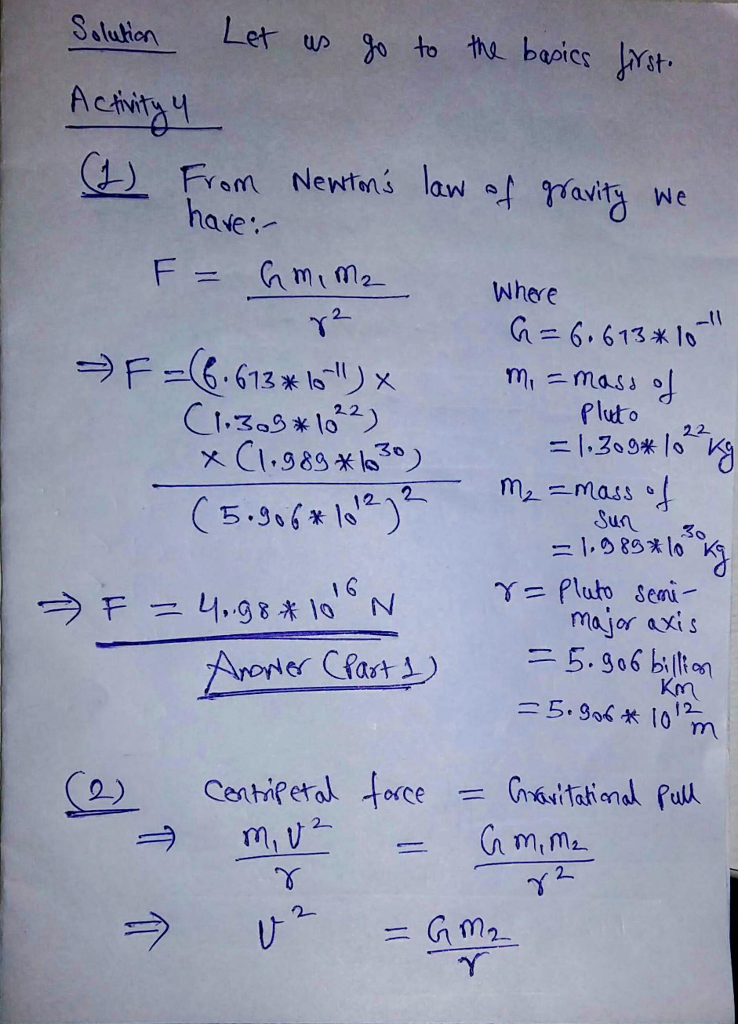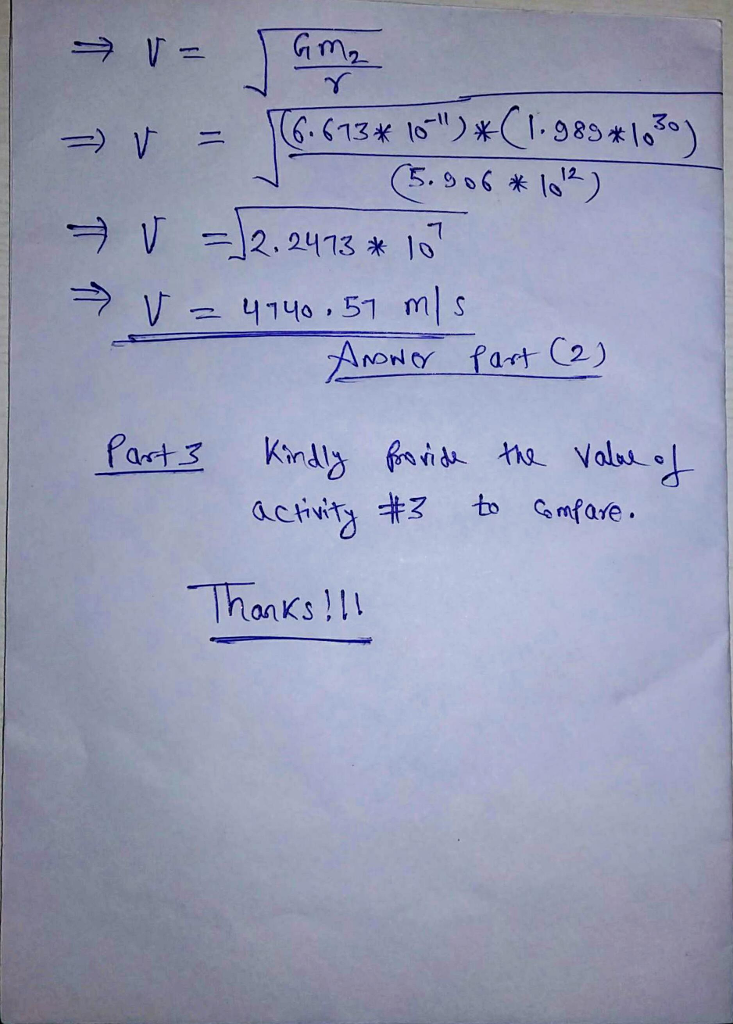Know the answer?
Your Answer:

#### Post as a guest

Your Name:

What's your source?

#### Earn Coin

Coins can be redeemed for fabulous gifts.

Not the answer you're looking for? Ask your own homework help question. Our experts will answer your question WITHIN MINUTES for Free.
Similar Homework Help Questions
• ### PLEASE HELP TO ANSWER THIS LAB ACTIVITY Activity 3: Kepler's Second Law: Objects in elliptical orbits...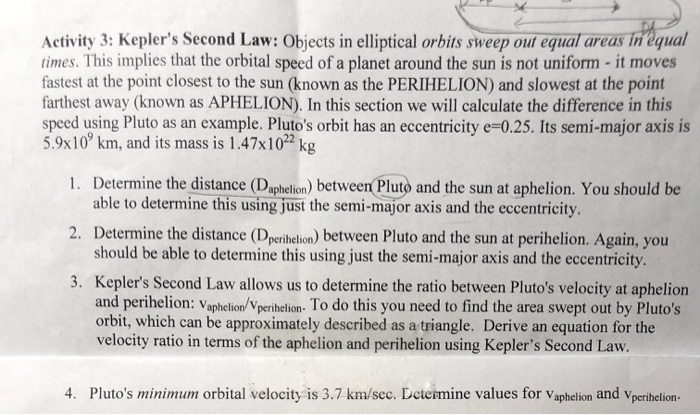PLEASE HELP TO ANSWER THIS LAB ACTIVITY Activity 3: Kepler's Second Law: Objects in elliptical orbits sweep oul equal areas in'equal times. This implies that the orbital speed of a planet around the sun is not uniform - it moves farthest away (known as APHELION). In this section we will calculate the difference in this fastest at the point closest to the sun (known as the PERIHELION) and slowest at the point speed using Pluto as an example. Pluto's orbit...

• ### Multivariable Calculus help with the magnitude of angular momentum: My questions is exercise 4 but I have attached exercise 1 and other notes that I was provided 4 Exercise 4. In any mechanics proble...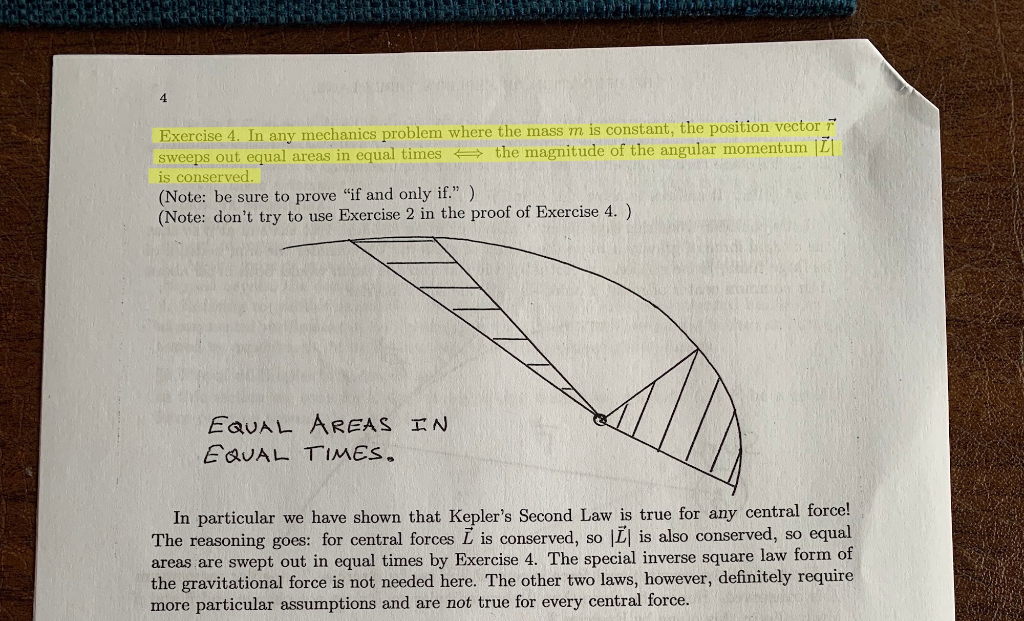Multivariable Calculus help with the magnitude of angular momentum: My questions is exercise 4 but I have attached exercise 1 and other notes that I was provided 4 Exercise 4. In any mechanics problem where the mass m is constant, the position vector F sweeps out equal areas in equal times the magnitude of the angular momentum ILI is conserved (Note: be sure to prove "if and only if") (Note: don't try to use Exercise 2 in the proof of...